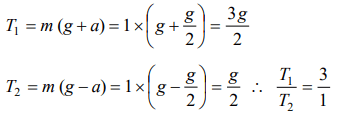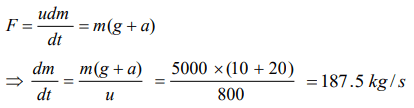## Laws of Motion Questions and Answers Part-5

1. The mass of a lift is 500 kg. When it ascends with an acceleration of $2 m\diagup s^{2}$ , the tension in the cable will be $\left[g=10 m\diagup s^{2}\right]$
a) 6000 N
b) 5000 N
c) 4000 N
d) 50 N

Explanation: T = m (g + a) = 500(10 + 2) = 6000 N

2. If force on a rocket having exhaust velocity of 300m/sec is 210 N, then rate of combustion of the fuel is
a) 0.7 kg/s
b) 1.4 kg/s
c) 0.07 kg/s
d) 10.7 kg/s

Explanation:3. In an elevator moving vertically up with an acceleration g, the force exerted on the floor by a passenger of mass M is
a) Mg
b) $\frac{1}{2}mg$
c) Zero
d) 2 Mg

Explanation: R = m (g +a) = m (g + g) = 2mg

4. A mass 1 kg is suspended by a thread. It is
(i) lifted up with an acceleration $4.9 m\diagup s^{2}$
(ii) lowered with an acceleration $4.9 m\diagup s^{2}$
The ratio of the tensions is
a) 3 : 1
b) 1 : 3
c) 1 : 2
d) 2 : 1

Explanation:5. A 5000 kg rocket is set for vertical firing. The exhaust speed is $800 m s^{-1}$ . To give an initial upward acceleration of $20 m s^{-2}$, the amount of gas ejected per second to supply the needed thrust will be $\left(g=10 ms^{-2}\right)$
a) $127.5 kg s^{-1}$
b) $187 .5 kg s^{-1}$
c) $185.5 kg s^{-1}$
d) $137.5 kg s^{-1}$

Explanation:6. If a person with a spring balance and a body hanging from it goes up and up in an aeroplane, then the reading of the weight of the body as indicated by the spring balance will
a) Go on increasing
b) Go on decreasing
c) First increase and then decrease
d) Remain the same

Explanation: Initially due to upward acceleration apparent weight of the body increases but then it decreases due to decrease in gravity.

7. The time period of a simple pendulum measured inside a stationary lift is found to be T. If the lift starts accelerating upwards with an acceleration g/3, the time period is
a) $T\sqrt{3}$
b) $T\sqrt{3}\diagup 2$
c) $T\diagup\sqrt{3}$
d) $T\diagup 3$

Explanation:8. A cork is submerged in water by a spring attached to the bottom of a pail. When the pail is kept in a elevator moving with an acceleration downwards, the spring length
a) Increases
b) Decreases
c) Remains unchanged
d) Data insufficient

Explanation:9. Two trolleys of mass m and 3m are connected by a spring. They were compressed and released once, they move off in opposite direction and comes to rest after covering distances $s_{1}$ and $s_{2}$ respectively. Assuming the coefficient of friction to be uniform, the ratio of distances $s_{1}:s_{2}$  is
a) 1 : 9
b) 1 : 3
c) 3 : 1
d) 9 : 1